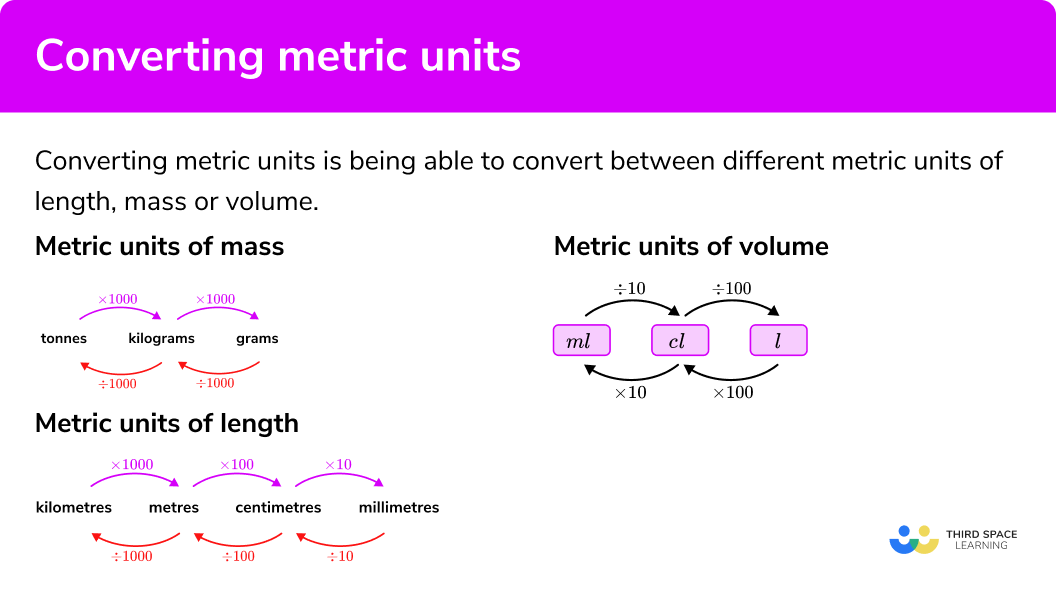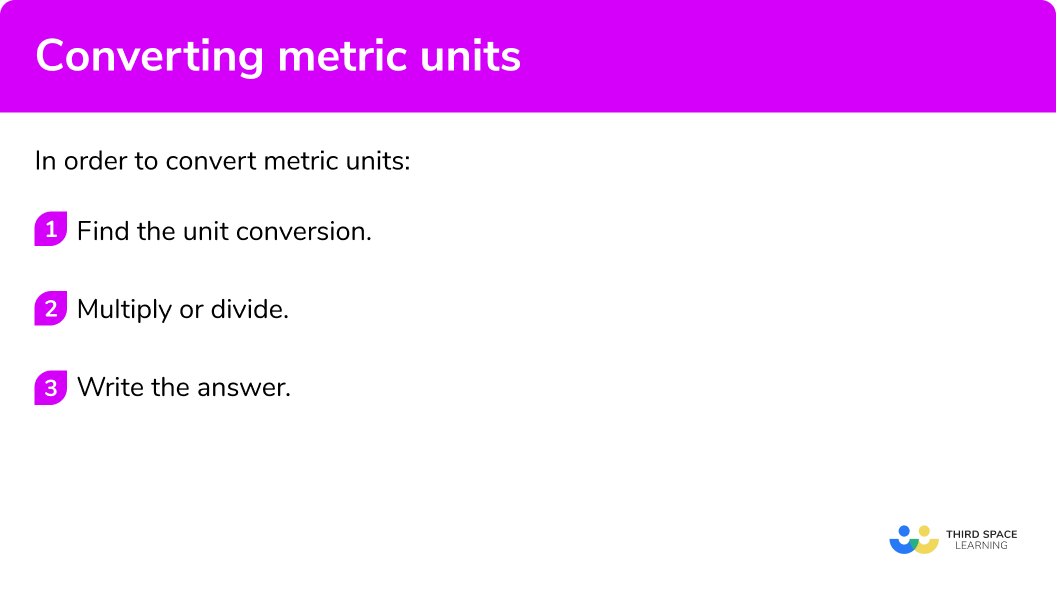# Converting Metric Units

Here we will learn about converting metric units, including metric units of length, metric units of mass and metric units of capacity (volume).

There are also converting metric units worksheets based on Edexcel, AQA and OCR exam questions, along with further guidance on where to go next if you’re still stuck.

## What is converting metric units?

Converting metric units is being able to convert between different metric units of length, mass or volume.

To do this we need to know what the metric units are and their conversion factors.

We can use prefixes to make these metric units bigger and smaller.

The main ones used are kilo, centi and milli:

### What is converting metric units?### Metric units of length

The SI unit (international system of units) of length is the metre (m) .

For length we mostly use kilometres (km) , metres (m) , centimetres (cm) and millimetres (mm) .

1 \ km=1000 \ m

1 \ m= 100 \ cm

1 \ m= 1000 \ mm

1 \ cm= 10 \ mm

E.g.

Covert 7 \ m to centimetres

7 \times 100=700

So, 7 \ m=700 \ cm

### Metric units of mass

The metric system for mass is based around grams (g) .

For mass we mostly use tonnes (t) , kilograms (kg) , and grams (g) .

1 \ tonne=1000 \ kg

1 \ kg= 1000 \ g

E.g.

Covert 4 \ kg to centimto grams

4 \times 1000=4000

So, 4 \ kg=4000 \ g

### Metric units of capacity (volume)

The SI unit The metric system for capacity is based on litres (l) .

For volume we mostly use litres (l) , centilites (cl) , and millilitres (ml) .

1 \ litre=1000 \ ml

1 \ litre= 100 \ cl

1 \ cl= 10 \ ml

We also need to be aware that:

1 \ litre=1000 \ cm^3

E.g.

Covert 6000 \ ml to litres

6000 \div 1000 = 6

So, 6000 \ ml=6 \ l

## How to convert metric units

In order to convert metric units:

1. Find the unit conversion.
2. Multiply or divide.

### How to convert metric units## Related lessons on units of measurement

Converting metric units is part of our series of lessons to support revision on units of measurement. You may find it helpful to start with the main units of measurement lesson for a summary of what to expect, or use the step by step guides below for further detail on individual topics. Other lessons in this series include:

## Converting metric units examples

### Example 1: converting length

Convert 3.2 \ m to cm

1. Find the unit conversion.

1 \ m=100 \ cm

2Multiply or divide.

As we are going from larger units to smaller units we multiply.

3.2 \times 100=320

3.2 \ m=320 \ cm

### Example 2: converting length

Convert 780 \ mm to cm

1 \ cm=10 \ mm

As we are going from smaller units to larger units we divide.

780 \div 10=78

780 \ mm=78 \ cm

### Example 3: converting mass

Convert 12.5 \ kg to g

1 \ kg=1000 \ g

As we are going from larger units to smaller units we multiply.

12.5 \times 1000=12 \ 500

12.5 \ kg=12 \ 500 \ g

### Example 4: converting mass

Convert 3800 \ g to kg

1 \ kg=1000 \ g

As we are going from smaller units to larger units we divide.

3800\div 1000=3.8

3800 \ g=3.8 \ kg

### Example 5: converting capacity

Convert 7.1 \ l to ml

1 \ l=1000 \ ml

As we are going from larger units to smaller units we multiply.

7.1 \times 1000=7100

7.1 \ l=7100 \ ml

### Example 6: converting capacity

Convert 750 \ ml to l

1 \ l=1000 \ ml

As we are going from smaller units to larger units we divide.

750 \div 1000=0.75

750 \ ml=0.75 \ l

### Example 7: converting capacity

Convert 6.8 \ l to cm^3

1 \ l=1000 \ cm^3

As we are going from larger units to smaller units we multiply.

6.8 \times 1000=6800

6.8 \ l=6800 \ cm^3

### Common misconceptions

• Multiply or divide?

Remember:

If you are going from larger units to smaller units – multiply
If you are going from smaller units to larger units – divide

### Practice converting metric units questions

1. Convert:  390 \ cm to m

39 \ m3.9 \ m390 \ m0.39 \ mConverting centimetres to metres we divide by 100 .

390\div 100=3.9 \ m

2. Convert:    57 \ cm to mm

5 \ 700 \ mm5.7 \ mm570 \ mm57 \ 000 \ mmConverting centimetres to millimetres we multiply by 10 .

57\times 10=570 \ cm

3. Convert:    81 \ 000 \ g to kg

810 \ kg81 \ kg8.1 \ kg8100 \ kgConverting grams to kilograms we divide by 1000 .

81\ 000\div 1000=81 \ kg

4. Convert:    630 \ kg to tonnes

6300 \ tonnes6.3 \ tonnes63 \ tonnes0.63 \ tonnesConverting kilograms to tonnes we divide by 1000 .

630\div 1000=0.63 \ tonnes

5. Convert:    4.8 \ l to ml

4800 \ ml480 \ ml48 \ ml48 \ 000 \ mlConverting litres to millilitres we multiply by 1000 .

4.8\times 1000=4800 \ ml

6. Convert:    560 \ ml to l

56 \ l0.56 \ l5.6 \ l0.056 \ lConverting millilitres to litres we divide by 1000 .

560\div 1000=0.56 \ l

### Converting metric units GCSE questions

1. Write 376 \ cm in metres.

(1 mark)

376\div 100=3.76

(1)

2. Here is a signpostHow far apart are the Town Centre and the Park?

(3 marks)

650\div 1000 = 0.65

Converting distance to km

(1)

3.5+0.65

(1)

4.15 \ km

(1)

3. A bottle contains 1.75 litres of lemonade.

A can contains 330 \ ml of lemonade.

How much lemonade does he have in total?

(3 marks)

1.75\times 1000=1750

Converting capacity to millilitres

(1)

2\times 1750+6\times 330

For finding the total

(1)

5480 \ ml

(1)

4.  4 tins of soup have a mass of 1.8kg.

3 tins of soup and 2 packets of soup have a mass of 1420 \ g.

Find the mass of 7 tins of soup and 5 packets of soup.

(5 marks)

1.8\div4=0.45 \ kg

Finding the mass of one tin

(1)

1420\div 1000=1.42 \ kg

Converting mass to kg

(1)

(1.42-3\times 0.45)\div 2=0.035

(1)

7\times 0.45+5\times 0.035

(1)

3.325 \ kg

(1)

## Learning checklist

You have now learned how to:

• Convert metric units of length
• Convert metric units of mass
• Convert metric units of capacity (volume)

## Still stuck?

Prepare your KS4 students for maths GCSEs success with Third Space Learning. Weekly online one to one GCSE maths revision lessons delivered by expert maths tutors.

Find out more about our GCSE maths tuition programme.